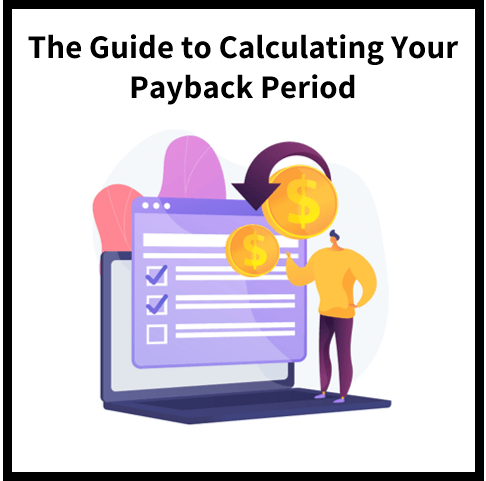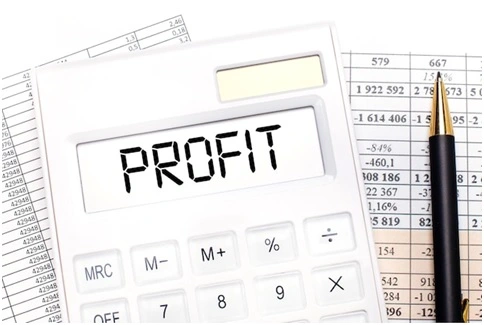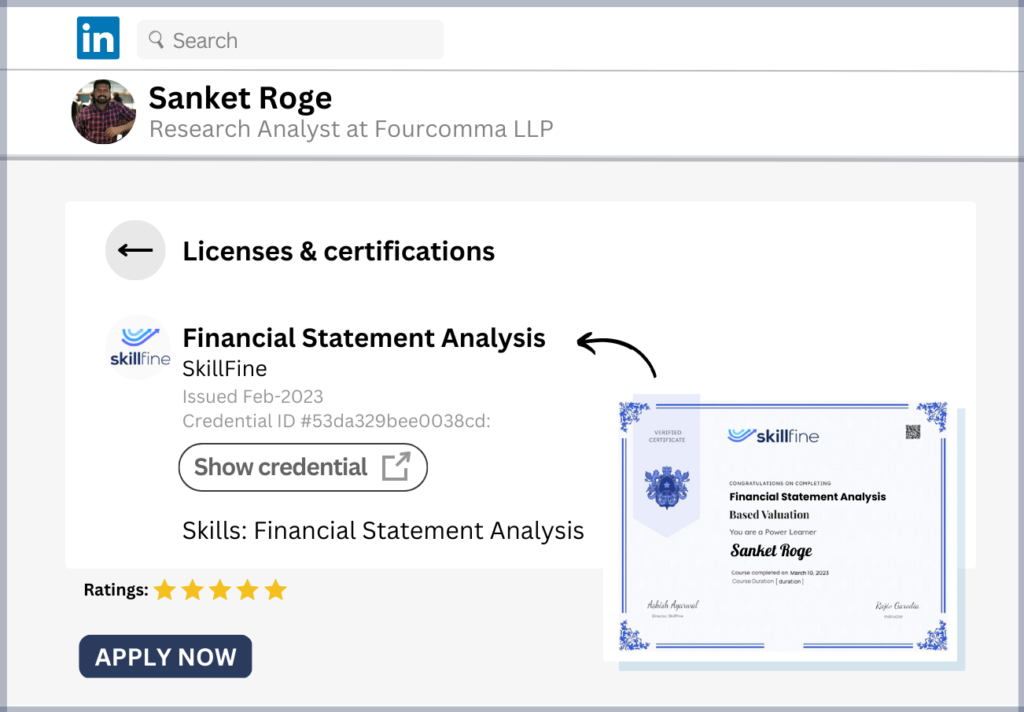# Calculating Your Payback Period: The Ultimate Guide## Calculating Your Payback Period: The Ultimate Guide

When evaluating an investment opportunity, you need to know how long it will take for your initial capital to be recouped. The payback period is the time it takes for a project to generate enough cash flow to recover its initial cost. Specifically, the payback period is the time required for the cash flows from operations (CFO) in a given accounting period to equal the initial capital investment.

In other words, the payback period is the length of time until an investor breaks even on their original capital expenditure. If the payback period is less than a year, then this signals that there’s good return on investment. On the other hand, if it’s over two years, then this signals that there may be something wrong with this business venture.

## What is the Payback Period?

The payback period is the amount of time it takes to recover the initial cost of an investment by collecting cash flows from that investment. The length of the payback period indicates whether an investment is worthwhile. If the payback period is less than one year, then the investor will break even on their investment in that time frame.

If it is longer than one year, then the investor will not break even until after one year. The payback period is a useful way to compare investments of different types. For example, if an investor is trying to decide between two projects, they can estimate each project’s payback period to determine which is more profitable. The project that has the shorter payback period is the better investment.### How to Calculate Payback Period

The payback period can be calculated as follows: Payback Period = Initial Investment / Cash Flow from Operations To understand this formula, let’s look at a real-life example. Imagine that you’re interested in purchasing a car. You’re trying to determine how long it will take to break even on that purchase.

First, you need to figure out how much the car will cost. Let’s say the car costs \$20,000. Next, you need to figure out how much you’ll be spending each year on the car. In this example, let’s say you plan to make monthly payments of \$500.

You’ll end up paying \$20,000 for the car after 36 months. Once you’ve calculated the time it will take for you to recover the initial cost of the car, you can use the payback period formula. The payback period in this example is 36 months. In other words, it takes 36 months for you to break even on your car purchase. Since this is less than a year, this indicates that purchasing this car is financially viable.

### Limitations of the Payback Period

The payback period has several limitations. First, it doesn’t account for future cash flows. Therefore, it doesn’t tell you how much money you’ll make in the long run. Second, it doesn’t account for the time value of money. In other words, it doesn’t take inflation into account. Third, it only calculates cash flows from operations.

As a result, it doesn’t account for other types of cash flows, like cash flow from financing. Finally, the payback period doesn’t take into account the risk involved with an investment. For example, an investment with a short payback period might be very risky. The investor might end up losing their initial capital.

An investment with a long payback period might be very safe. In other words, the investor might never lose their initial capital. The payback period doesn’t account for these differences in risk.

### Why the Payback Period is Important

The payback period is an important concept in finance because it helps investors make sound decisions about whether or not to invest in an opportunity. It’s important to understand the length of time until you break even on an investment so that you don’t lose money. If an investment has a short payback period, you’ll break even more quickly. This means you’ll have more money to put towards other investments.

Similarly, it’s important to know the length of time until you break even because it determines how much risk you’re exposed to. Investments with a long payback period have less risk than those with a short payback period. This is because you won’t lose your initial capital as quickly.

### Limitations of calculating payback period

The most significant limitation of the payback period is that it does not take into account the time value of money. In other words, it does not take inflation into account. For example, let’s say you’re evaluating two different investment opportunities.

One has a short payback period, while the other has a long payback period. The short payback period investment might seem like a more attractive option. After all, you’ll break even on your initial capital more quickly. However, you have to account for inflation. Inflation is when the general price level of goods and services rise over time. As a result, the value of your money falls as time goes by.

### Using Net Present Value to find the payback period

If you’re evaluating different types of investments, you can use the Net Present Value (NPV) to find the payback period. The NPV measures the profitability of an investment by calculating the present value of all cash flows generated by an investment. In other words, it takes into account your initial capital as well as the time value of money.

The NPV is calculated as follows: Net Present Value = (Cash Flow from Operations * Discount Rate) – Initial Capital Investment Once you’ve calculated the NPV for each investment, you can use the formula above to calculate the payback period. As a result, you’ll be able to compare the profitability of each investment. The investment with the highest NPV has the highest profitability.

### Using Return on Investment to find the payback period

Alternatively, you can use the Return on Investment (ROI) to find the payback period. The ROI is calculated as follows: Return on Investment = (Cash Flow from Operations / Initial Capital Investment) * 100 This formula tells you how much money you’re making on each dollar you invest.

For example, if you have an investment that earns \$5 on every dollar you invest, this would give you an ROI of 500%. Once you’ve calculated the ROI for each investment, you can use it to find the payback period. This is because the ROI can be used to calculate the payback period. You can calculate the ROI for each investment, then use the payback period formula above to find the length of time until you break even on those investments.

### Bottom line

The payback period is a simple metric that allows you to quickly compare different investment opportunities. It tells you the length of time until you break even on an investment. The shorter the payback period, the better the investment. The payback period is only useful when comparing investments of the same type.

In other words, you can only use it to compare different types of cash flows. It cannot be used to compare investments with different types of cash flows. Additionally, the payback period only tells you about how long it will take to break even on an investment. It does not tell you anything about the long-term viability of an investment. In other words, it does not account for the time value of money.

### Related Courses: Credit Analysis Skills Training Courses